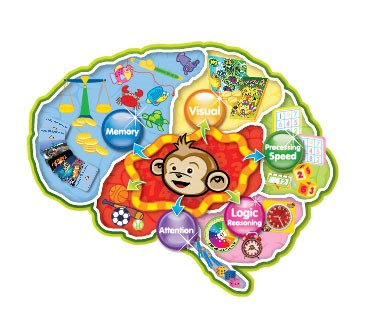## Math Monkey helps your child to develop MathBrain

"Our job is to develop a MathBrain in your child."At Math Monkey, we understand that the human brain has a natural learning process: Curious> Explore> Test> Repeat> Master.

Therefore, we pay more attention to ensure that the learning process is comprehensive. We empower your child to have the courage to learn from experiences and to have the initiative to explore new knowledge. As they learn in this manner, they will eventually form the habit of an independent learner. This way of learning can further improve the 5 major brain abilities: attention, visual, memory, logical thinking and processing speed, resulting in a MathBrain!

For example:

1. In order for a child to process 7 + 8, the mental process requires

(i) the recognition of the numbers,
(ii) the ability to picture what these numbers quantitatively represent,
(iii & iv) logically put the two numbers together in order to arrive at a probable answer, e.g. 7 + 8 = 20
(this would be illogical because the answer would be too big), and finally
(v) how long it takes for the child to complete the calculation; the time required would need to be within reason.

2. What is “half of 36?” This question would not be one that a child can have a memorized answer. This would require a child to mentally solve the problem by

(i) recognition of 36 in 2 parts, 30 and 6
(ii) knowing quantitatively what 30 and 6 represent,
(iii & iv) remembering these two numbers and the halves of each,
(v) to do it within a reasonable amount of time.